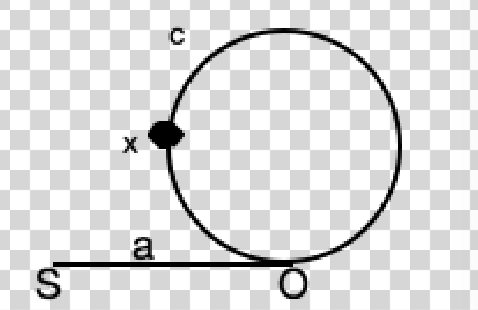# 287. Find the Duplicate Number 寻找重复数

@TOC

## # 题目描述

Given an array nums containing n + 1 integers where each integer is between 1 and n (inclusive), prove that at least one duplicate number must exist. Assume that there is only one duplicate number, find the duplicate one.

Example 1:

``````Input: [1,3,4,2,2]
Output: 2
``````

Example 2:

``````Input: [3,1,3,4,2]
Output: 3
``````

Note:

1. You must not modify the array (assume the array is read only).
2. You must use only constant, O(1) extra space.
3. Your runtime complexity should be less than O(n2).
4. There is only one duplicate number in the array, but it could be repeated more than once.

## # 解题方法

### # 保存已经访问过的数字

C++代码如下：

``````class Solution {
public:
int findDuplicate(vector<int>& nums) {
unordered_set<int> visited;
for (int num : nums) {
if (visited.count(num))
return num;
visited.insert(num);
}
return -1;
}
};
``````

### # 链表成环1. 首先要证明的是，两指针相遇时，慢指针还没有走完整个链表。

• 当慢指针没走完一圈时，显然成立
• 假设慢指针走完了一圈之后相遇，可以假定快指针在O的前一个位置，慢指针走一圈回到了O点，此时快指针走了两圈又回到了O的前一个位置，所以在慢指针走玩一圈之前就已经相遇。
2. 快慢指针在x处第一次汇合，xo之间距离为x，假如快指针走了n圈，快指针走过的路程为a+x+n*(c + x)，慢指针走过的路程为a+x，所以a+x+n*(c + x) = 2(a+x),所以a + x = n*(c + x)，也就是SOx之间的距离等于n圈的长度，所以令快指针从起点开始一次一步，慢指针从x开始顺时针方向转，同时前进，则快指针走a时，慢指针走了n*(c+x) - x的长度，则必会在O处相遇！

``````class Solution(object):
def findDuplicate(self, nums):
"""
:type nums: List[int]
:rtype: int
"""
slow = nums
fast = nums[nums]
while slow != fast:
fast = nums[nums[fast]]
slow = nums[slow]
fast = 0
while slow != fast:
fast = nums[fast]
slow = nums[slow]
return fast
``````

C++解法如下：

``````class Solution {
public:
int findDuplicate(vector<int>& nums) {
const int N = nums.size();
int slow = nums, fast = nums[nums];
while (fast != slow) {
fast = nums[nums[fast]];
slow = nums[slow];
}
fast = 0;
while (fast != slow) {
fast = nums[fast];
slow = nums[slow];
}
return fast;
}
};
``````

### # 二分查找

``````class Solution {
public:
int findDuplicate(vector<int>& nums) {
const int N = nums.size();
int l = 0, r = N;
// [l, r)
while (l < r) {
int mid = l + (r - l) / 2;
int count = 0;
for (int n : nums){
if (n <= mid) {
count ++;
}
}
if (count <= mid)
l = mid + 1;
else
r = mid;
}
return r;
}
};
``````

http://blog.csdn.net/monkeyduck/article/details/50439840 https://blog.csdn.net/l294265421/article/details/50478818

## # 日期

2018 年 3 月 12 日 2018 年 12 月 23 日 —— 周赛成绩新高 2019 年 1 月 11 日 —— 小光棍节？